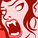# New to Qlik Sense

If you’re new to Qlik Sense, start with this Discussion Board and get up-to-speed quickly.

Announcements
QlikWorld 2023, a live, in-person thrill ride. Save \$300 before February 6: REGISTER NOW!
cancel
Showing results for
Did you mean:Creator III

## Number of months are not coming post 11, But I want to show 24 months comparison

Hi All, I have this requirement where we have to show the requests inflowed 24 months before the contract end date. but the below expression that i have written only showing the counts till 12 Months in the bar chart with numbers from 0 to 11.

below is the dimension expression am using :

=

if(Inflow_Month = Month(Contract_End_Date)-24 , 24,

if(Inflow_Month = Month(Contract_End_Date)-23 , 23,

if(Inflow_Month = Month(Contract_End_Date)-22, 22,

if(Inflow_Month = Month(Contract_End_Date)-21, 21,

if(Inflow_Month = Month(Contract_End_Date)-20 , 20,

if(Inflow_Month = Month(Contract_End_Date)-19 , 19,

if(Inflow_Month = Month(Contract_End_Date)-18 , 18,

if(Inflow_Month = Month(Contract_End_Date)-17, 17,

if(Inflow_Month = Month(Contract_End_Date)-16,16,

if(Inflow_Month = Month(Contract_End_Date)-15, 15,

if(Inflow_Month = Month(Contract_End_Date)-14 , 14,

if(Inflow_Month = Month(Contract_End_Date)-13 , 13,

if(Inflow_Month = Month(Contract_End_Date)-12 , 12,

if(Inflow_Month = Month(Contract_End_Date)-11 , 11,

if(Inflow_Month = Month(Contract_End_Date)-10 , 10,

if(Inflow_Month = Month(Contract_End_Date)-9 ,9,

if(Inflow_Month = Month(Contract_End_Date)-8, 8,

if(Inflow_Month = Month(Contract_End_Date)-7 , 7,

if(Inflow_Month = Month(Contract_End_Date)-6 , 6,

if(Inflow_Month = Month(Contract_End_Date)-5 , 5,

if(Inflow_Month = Month(Contract_End_Date)-5 , 4,

if(Inflow_Month = Month(Contract_End_Date)-3 ,3,

if(Inflow_Month = Month(Contract_End_Date)-2 , 2,

if(Inflow_Month = Month(Contract_End_Date)-1 , 1,

if(Inflow_Month = Month(Contract_End_Date), 0))))))))))))))

in the below chart it is showing just 12 months instead i want this to display 24 monthsV.

Labels (5)

• ### Visualization

2 RepliesSpecialist II

for 13 you have define like below:

= if(Inflow_Month = Num(Contract_End_Date)-13 , 13,

there it should be Month or Num?Creator III
Author

Hi thanks edited correctly now, still the formula is not working. it could be because of the when we write month() max it  will take till 12 only.

Any one suggest the right way to achieve it please.Tags
Community Browser